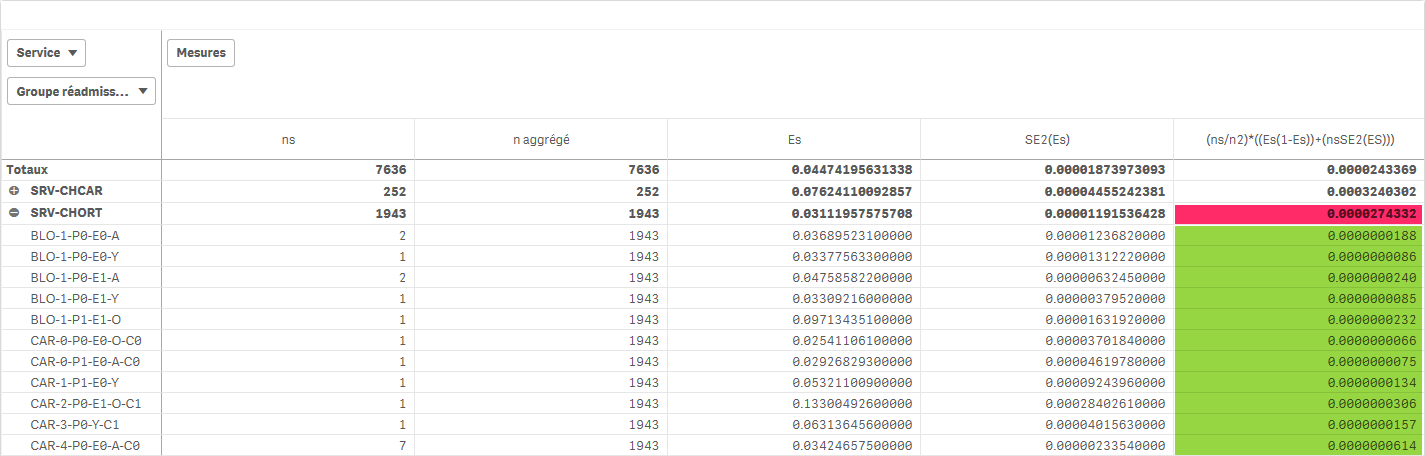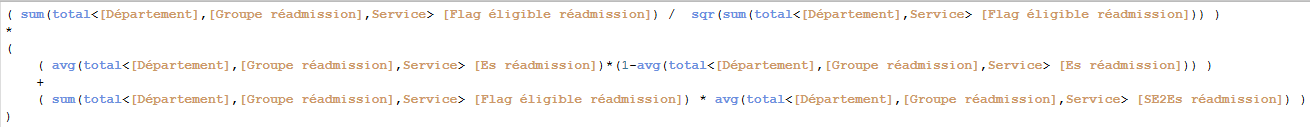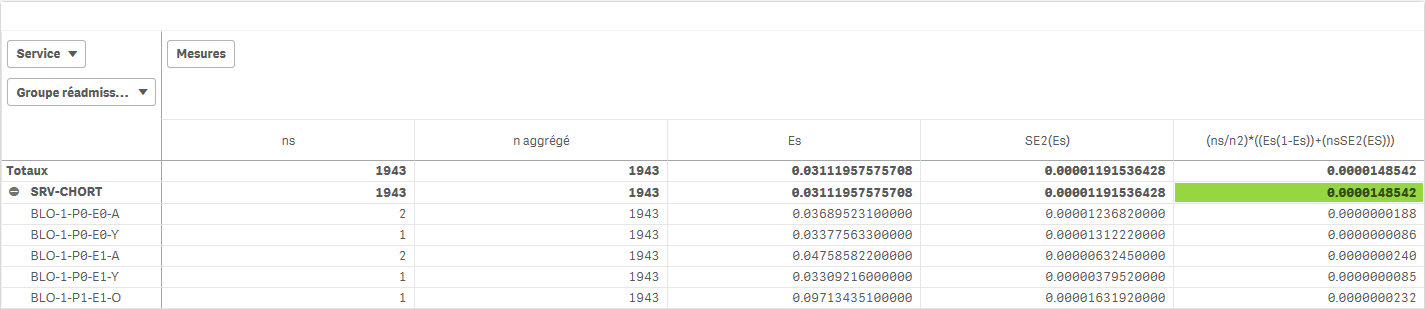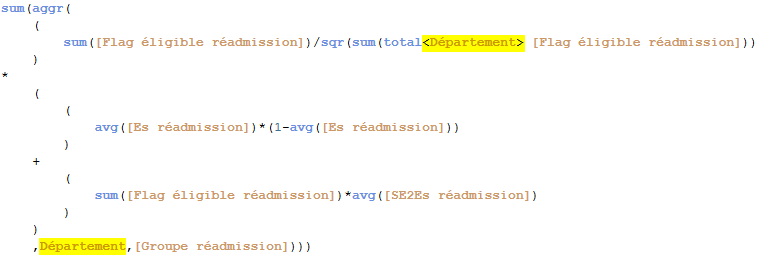# Qlik Sense App Development

Announcements
BARC’s The BI Survey 19 makes it official. BI users love Qlik. GET REPORT
Highlighted
New Contributor II

## Tricky calculations agregations

Hi all,

I try to implement a complex statistic calculation (trust interval) but I fail on an agregation problem :Calculation of the (ns/n2)*((Es(1-Es))+(nsSE2(ES))) column should be done at Groupe réadmission level and should be able to be agregated on all others higher levels, like, here, Service or Département.

Calculation at Groupe réadmission level is ok (in green) and can be automatically agregated but it's impossible to get the right sum at Service level. Result is always wrong (in red).

Here is the formula of the (ns/n2)*((Es(1-Es))+(nsSE2(ES))) column :If I do what is the most logic for me : sum(aggr( <the_formula> ,[Groupe réadmission])) then result is different but still wrong :It's correct only if a single Service is selected :I don't understand. I probably miss something. If someone has an idea, I would be happy to get it.

Stéphane

1 Solution

Accepted Solutions
New Contributor II

## Re: Tricky calculations agregations

Thank you Sunny.

Yes, you're right. Actually, I tried this but difficulty was that a part of the formula has to be summed over all the dimension.

Finally, I succeeded by adding the upper dimension on witch the sum has to be done in the context and by adding it also on the TOTAL option :Unfortunatly, this formula can not be agregated on other dimension than the one which is specified so I have to multiply the expressions to have always the one to match where I want to use it. Here is the formula for the Département graph, if I want to make a graph per Service then I have to rewrite another expression with Service instead of Département but ok, that works like that.

Of course, if anyone has a better idea, I would be happy to know it.

Stéphane

4 RepliesMVP

## Re: Tricky calculations agregations

You need to use Sum(Aggr(YourExpression, YourDimensions)) to fix this... also check here

Sum of rows in pivot tables ‒ QlikView

Totals in Charts

New Contributor II

## Re: Tricky calculations agregations

Thank you Sunny.

Yes, you're right. Actually, I tried this but difficulty was that a part of the formula has to be summed over all the dimension.

Finally, I succeeded by adding the upper dimension on witch the sum has to be done in the context and by adding it also on the TOTAL option :Unfortunatly, this formula can not be agregated on other dimension than the one which is specified so I have to multiply the expressions to have always the one to match where I want to use it. Here is the formula for the Département graph, if I want to make a graph per Service then I have to rewrite another expression with Service instead of Département but ok, that works like that.

Of course, if anyone has a better idea, I would be happy to know it.

StéphaneMVP

## Re: Tricky calculations agregations

I think what you have is probably the best you can do.

New Contributor II

## Re: Tricky calculations agregations

I think so too. Thanks anyway Sunny.

Talk to you. Bye !

Stéphane